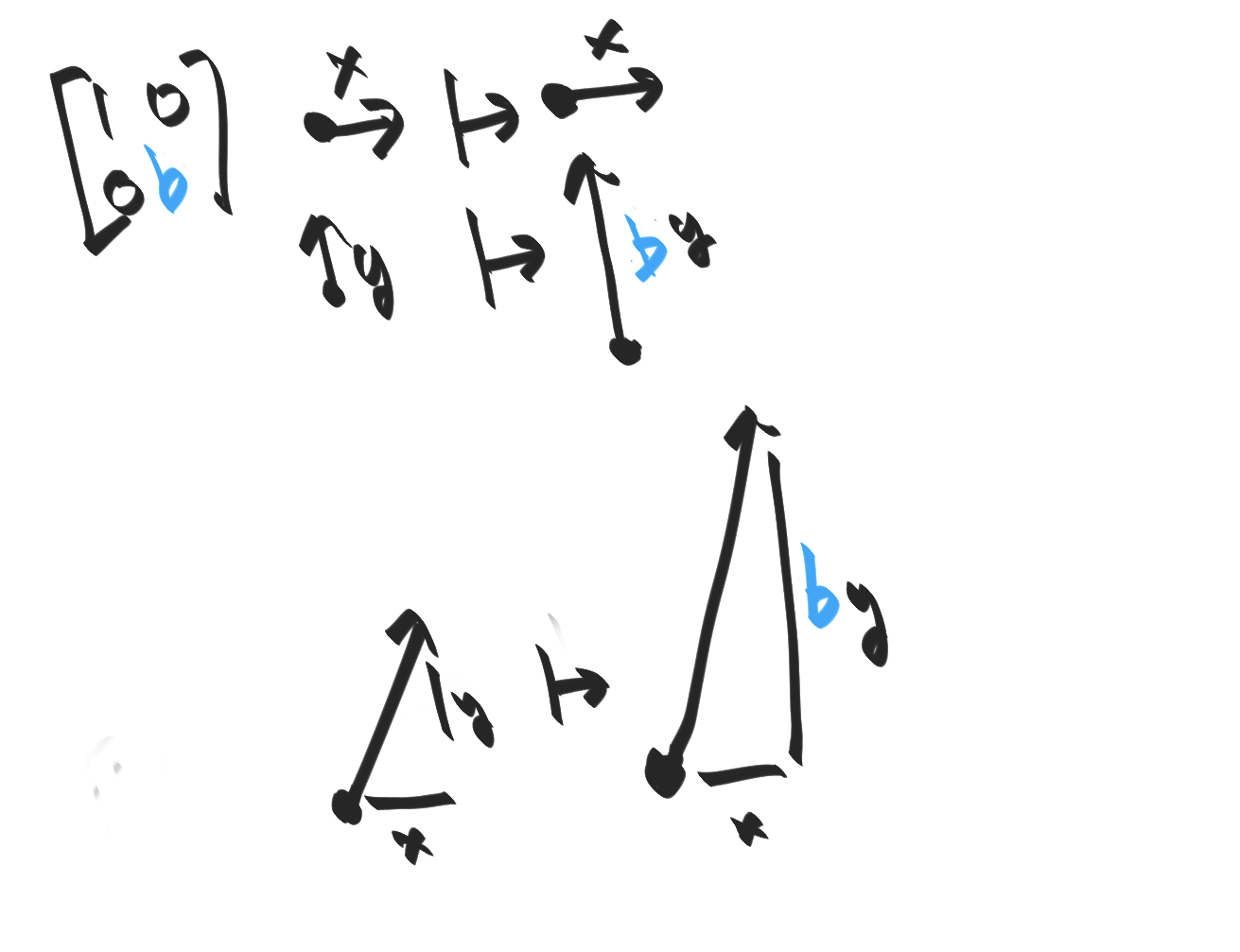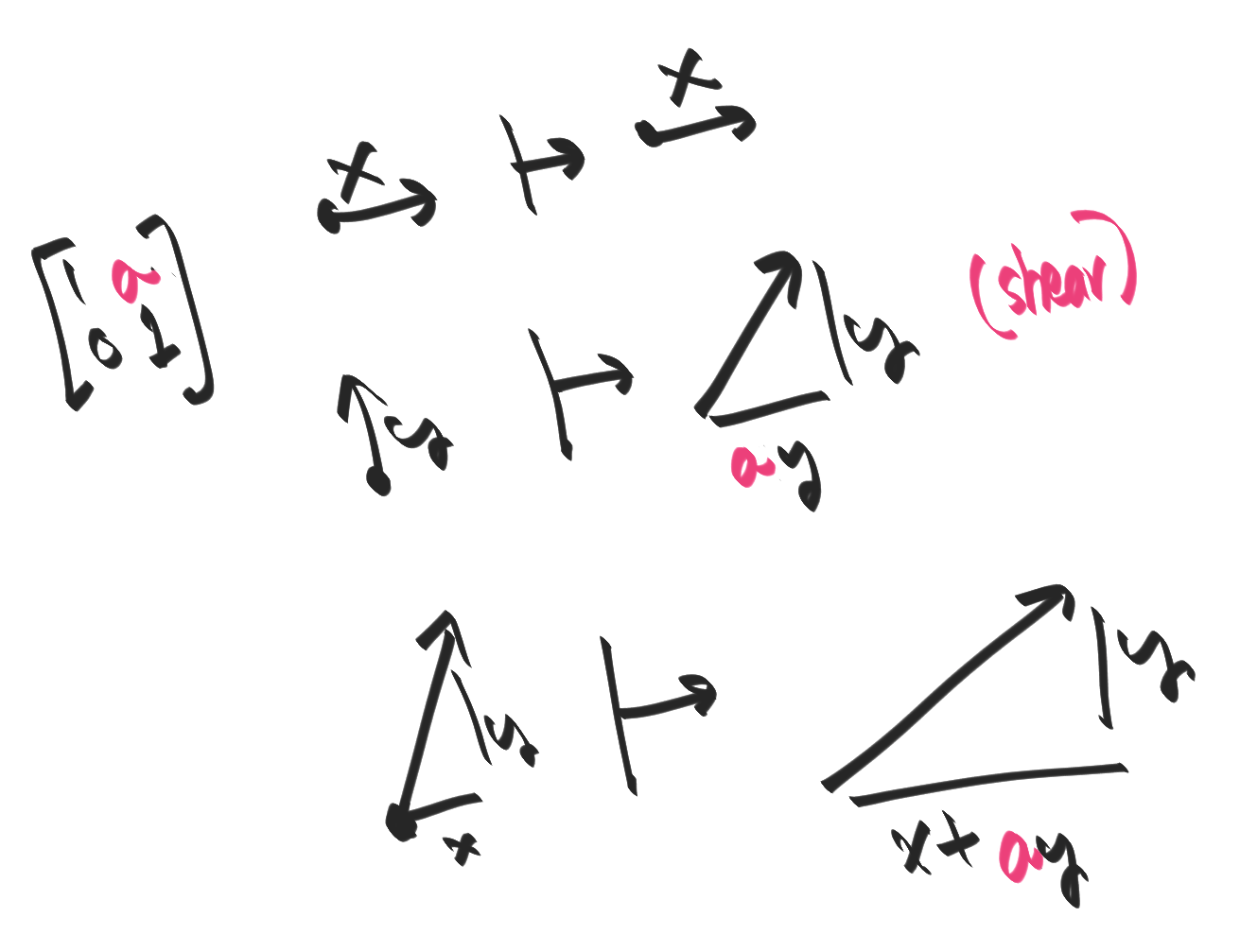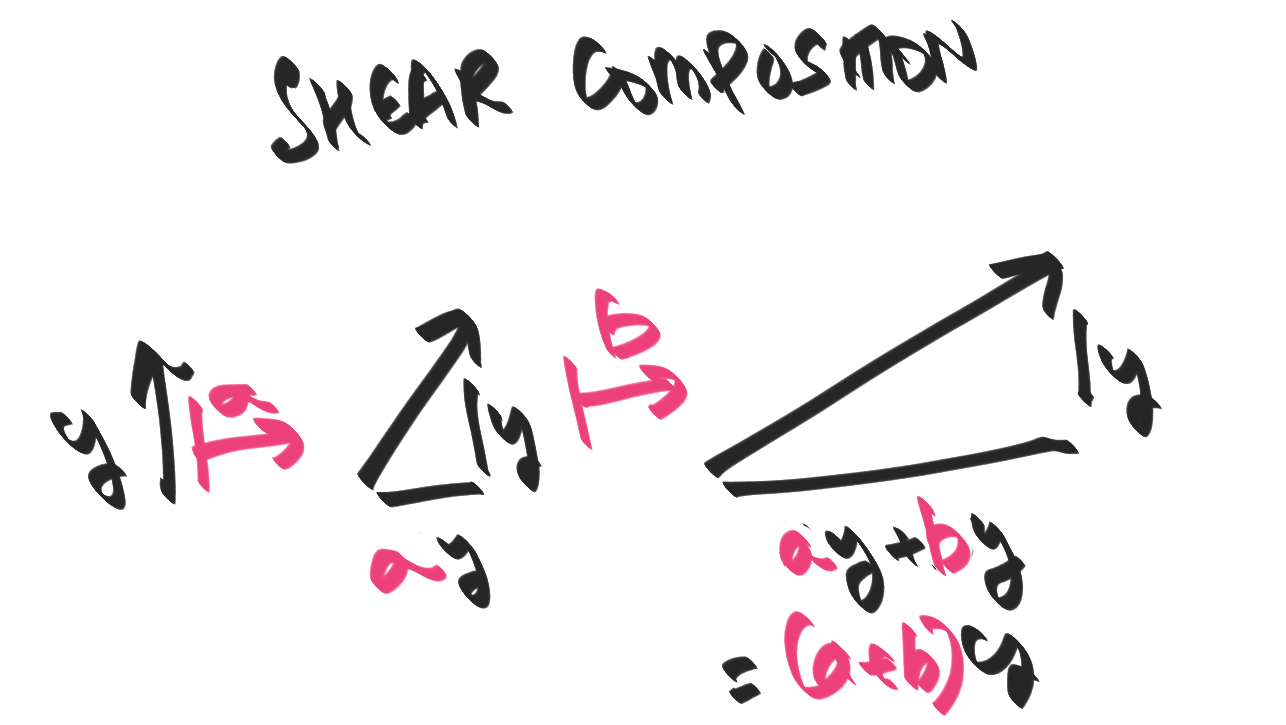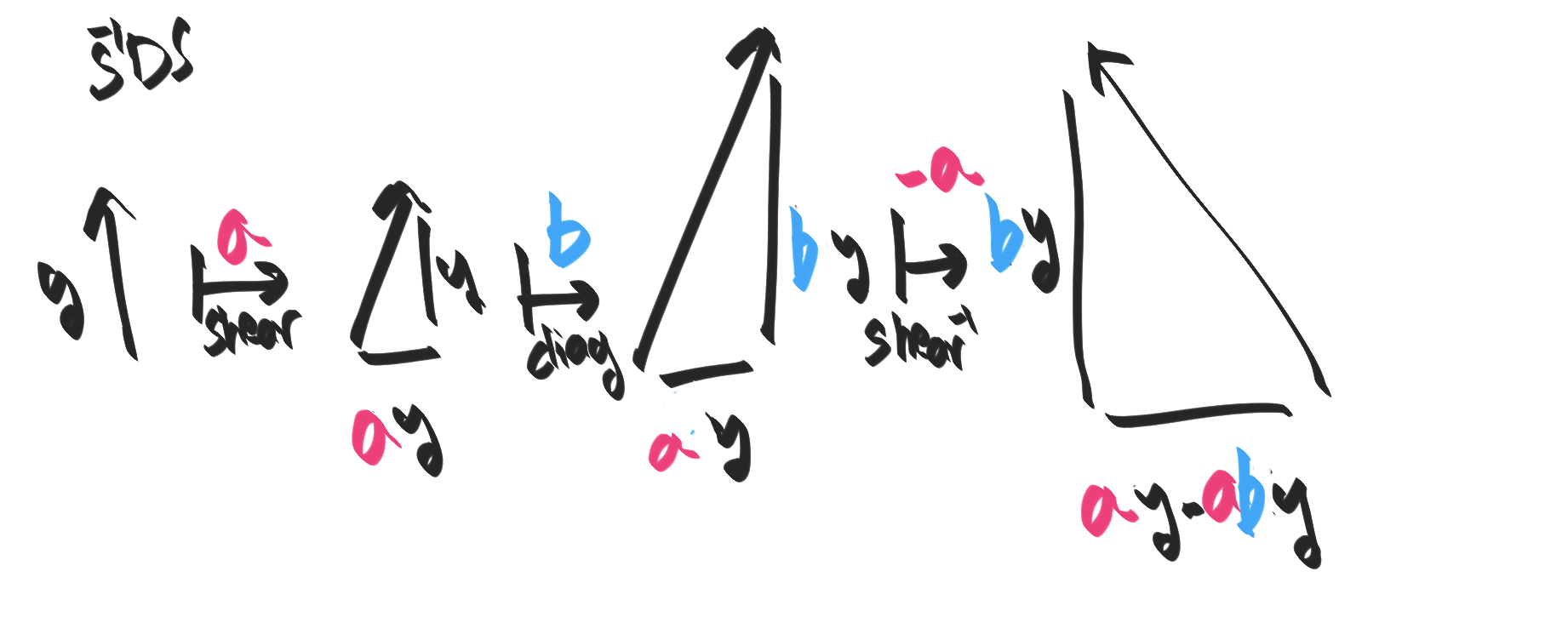## § A semidirect product worked on in great detail

We work out the semidirect product structure of the collection of real 2x2 matrices
$\begin{bmatrix} 1 & a \\ 0 & b \end{bmatrix}$
We first see that the multiplication rule is:
$\begin{bmatrix} 1 & a \\ 0 & b \end{bmatrix} \begin{bmatrix} 1 & p \\ 0 & q \end{bmatrix} = \begin{bmatrix} 1 & p + bq \\ 0 & bq \end{bmatrix}$
so these are closed under matrix multiplication. The identity matrix is one among these matrices and thus we have the identity. The inverse of such a matrix can also be seen to be of such a kind.

#### § Diagonal transforms

We have two subgroups of matrices in this set of 2x2 matrices. The first of these I shall call diagonal and denote with $D$:
$\begin{bmatrix} 1 & 0 \\ 0 & b \end{bmatrix} \begin{bmatrix} 1 & 0 \\ 0 & q \end{bmatrix} = \begin{bmatrix} 1 & 0 \\ 0 & bq \end{bmatrix}$Hopefully clearly, this is isomorphic to $\mathbb R^*$ since the only degree of freedom is the bottom right entry, which gets multiplied during matrix multiplication. These transform a vector $(x, y)$ into the vector $(x, \delta y)$. Informally, the $D$ matrices are responsible for scaling the $y$-axis.#### § Shear transforms

Next, we have the other subgroup of matrices, which I shall call shear and denote by $S$:
$\begin{bmatrix} 1 & a \\ 0 & 1 \end{bmatrix} \begin{bmatrix} 1 & p \\ 0 & 1 \end{bmatrix} = \begin{bmatrix} 1 & (a+p) \\ 0 & 1 \end{bmatrix}$These are isomorphic to $\mathbb R^+$, since the only degree of freedom is their top-right entry, which gets added on matrix multiplication. These matrices transform a vector $(x, y)$ into $(x + \delta y, y)$.#### § Generating all transforms with diagonal and shear transforms

we can write any transform of the form
$T \equiv \begin{bmatrix} 1 & a \\ 0 & b \end{bmatrix}$

#### § Semidirect product: Conjugations

We need to check whether the subgroup $D$ or the subgroup $S$ is normal. For this, take two arbitrary elements:
$[d] \equiv \begin{bmatrix} 1 & 0 \\ 0 & d \end{bmatrix}; ~~~ [s] \equiv \begin{bmatrix} 1 & s \\ 0 & 1 \end{bmatrix}$

#### § Conjugating $D$ by $S$:

Let's to conjugate a diagonal with a shear:
\begin{aligned} &[s^{-1}][d][s](x, y) \\ &= [s^{-1}][d] (x+sy, y) \\ &= [s^{-1}](x+sy, dy) \\ &= (x+sy-sdy, dy) \\ \end{aligned}
This doesn't leave us with another diagonal transform.#### § Conjugating $S$ with $D$

Now let's compute the action of $dsd^{-1}$, and $sds^{-1}$ on some general $(x, y)$:
\begin{aligned} &[d^{-1}][s][d](x, y) \\ &= [d^{-1}][s] (x, dy) \\ &= [d^{-1}](x + dy, dy) \\ &= [d^{-1}](x + dy, dy \times 1/d \\ &= (x + dy, y) \end{aligned}
See that the final result we end up with is a shear transform which shears by $y/d$. So, we can write the equation $DSD^{-1} = S$: conjugating a shear by scaling leaves us with a shear.#### § The connnection to partial fractions

Recall that any matrix of the form [a b; c d] can be viewed as taking the fraction x/y to (ax+by)/(cx+dy). In our case, we have:
• Diagonal: [1 0; 0 b] which take x/y to x/by.
• Shear: [1 a 0 1] which take x/y to (x + ay)/y.
It's clear that diagonals and shears compose. What is unclear is how they interact. A little thought shows us:
x/y -diagonal->
x/dy -shear->
(x+sdy)/dy
(x+sy')/y'

x/y -shear->
(x+sy)/y -diagonal->
(x+sy)/dy
= (x+(s/d)y')/y'

So, when we compse shears with diagonals, we are left with "twisted shears". The "main objects" are the shears (which are normal), and the "twists" are provided by the diagonal. The intuition for why the twisted obect (shears) should be normal is that the twisting (by conjugation) should continue to give us twisted objects (shears). The "only way" this can resonably happen is if the twisted subgroup is normal: ie, invariant under all twistings/conjugations.

#### § How the semidirect product forms

From the above computations, we can see that it is the shear transform $S$ that are normal in the collection of matrices we started out with, since $D^{-1}SD = S$. Intuitively, this tells us that it is the diagonal part of the transform composes normally, and the shear part of the transform is "twisted" by the diagonal/scaling part. This is why composing a shear with a diagonal (in either order --- shear followed by diagonal or vice versa) leaves us with a twisted shear. This should give a visceral sense of "direct product with a twist".

#### § Where to go from here

In some sense, one can view all semidirect products as notationally the same as this example so this example provides good intuition for the general case.